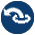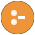#solution_sequences.pl -- Modify solution sequences

The meta predicates of this library modify the sequence of solutions of a goal. The modifications and the predicate names are based on the classical database operations DISTINCT, LIMIT, OFFSET, ORDER BY and GROUP BY.

These predicates were introduced in the context of the SWISH Prolog browser-based shell, which can represent the solutions to a predicate as a table. Notably wrapping a goal in distinct/1 avoids duplicates in the result table and using order_by/2 produces a nicely ordered table.

However, the predicates from this library can also be used to stay longer within the clean paradigm where non-deterministic predicates are composed from simpler non-deterministic predicates by means of conjunction and disjunction. While evaluating a conjunction, we might want to eliminate duplicates of the first part of the conjunction. Below we give both the classical solution for solving variations of (`a(X)`, `b(X)`) and the ones using this library side-by-side.

Avoid duplicates of earlier steps
```  setof(X, a(X), Xs),               distinct(a(X)),
member(X, Xs),                    b(X)
b(X).```

Note that the distinct/1 based solution returns the first result of `distinct(a(X))` immediately after a/1 produces a result, while the setof/3 based solution will first compute all results of a/1.

Only try `b(X)` only for the top-10 `a(X)`
```  setof(X, a(X), Xs),               limit(10, order_by([desc(X)], a(X))),
reverse(Xs, Desc),                b(X)
first_max_n(10, Desc, Limit),
member(X, Limit),
b(X)```

Here we see power of composing primitives from this library and staying within the paradigm of pure non-deterministic relational predicates.

- all solution predicates findall/3, bagof/3 and setof/3.
- library(aggregate)
distinct(:Goal)
distinct(?Witness, :Goal)
True if Goal is true and no previous solution of Goal bound Witness to the same value. As previous answers need to be copied, equivalence testing is based on term variance (=@=/2). The variant distinct/1 is equivalent to `distinct(Goal,Goal)`.

If the answers are ground terms, the predicate behaves as the code below, but answers are returned as soon as they become available rather than first computing the complete answer set.

```distinct(Goal) :-
findall(Goal, Goal, List),
list_to_set(List, Set),
member(Goal, Set).```
reduced(:Goal)
reduced(?Witness, :Goal, +Options)
Similar to distinct/1, but does not guarantee unique results in return for using a limited amount of memory. Both distinct/1 and reduced/1 create a table that block duplicate results. For distinct/1, this table may get arbitrary large. In contrast, reduced/1 discards the table and starts a new one of the table size exceeds a specified limit. This filter is useful for reducing the number of answers when processing large or infinite long tail distributions. Options:
size_limit(+Integer)
Max number of elements kept in the table. Default is 10,000.
limit(+Count, :Goal)
Limit the number of solutions. True if Goal is true, returning at most Count solutions. Solutions are returned as soon as they become available.
Arguments:
 Count - is either `infinite`, making this predicate equivalent to call/1 or an integer. If Count < 1 this predicate fails immediately.
offset(+Count, :Goal)
Ignore the first Count solutions. True if Goal is true and produces more than Count solutions. This predicate computes and ignores the first Count solutions.
call_nth(:Goal, ?Nth)
True when Goal succeeded for the Nth time. If Nth is bound on entry, the predicate succeeds deterministically if there are at least Nth solutions for Goal.
order_by(+Spec, :Goal)
Order solutions according to Spec. Spec is a list of terms, where each element is one of. The ordering of solutions of Goal that only differ in variables that are not shared with Spec is not changed.
asc(Term)
Order solution according to ascending Term
desc(Term)
Order solution according to descending Term
group_by(+By, +Template, :Goal, -Bag) is nondet
Group bindings of Template that have the same value for By. This predicate is almost the same as bagof/3, but instead of specifying the existential variables we specify the free variables. It is provided for consistency and complete coverage of the common database vocabulary.

## Undocumented predicates

The following predicates are exported, but not or incorrectly documented.

reduced(Arg1, Arg2, Arg3)
distinct(Arg1, Arg2)